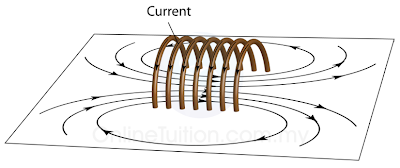# Magnetic Effects of a Current-Carrying Conductor - Solenoid

A solenoid is a long coil made up of a numbers of turns of wire.

Magnetic Field Pattern

1. Figure (a) illustrates the field pattern produced by a solenoid when current pass through it.
2. The field lines in the solenoid are close to each other, indicates that the magnetic field is stronger inside the solenoid.
3. We can also see that the field lines are parallel inside the solenoid. This shows that the strength of the magnetic filed is about uniform inside the solenoid.
4. We can also see that the magnetic field of a solenoid resembles that of the long bar magnet, and it behaves as if it has a North Pole at one end and a South Pole at the other.(Figure (a): Magnetic field pattern of a solenoid)

Determining the Pole of the Magnetic Field

1. The pole of the magnetic field of a solenoid can be determined by the Right Hand Grip Rule.
2. Imagine your right-hand gripping the coil of the solenoid such that your fingers point the same way as the current. Your thumb then points in the direction of the field.
3. Since the magnetic field lines always come out from the North Pole, hence the thumb points towards the North Pole.

1. There is another method can be used to determine the poles of the magnetic field forms by a solenoid.
2. Try to visualise that you are viewing the solenoid from the 2 ends as illustrated in figure (c) below.
3. The end will be a North pole if the current is flowing in the aNticlockwise, or a South pole if the current is flowing in the clockwiSe direction.Strength of the Magnetic Field
The strength of the magnetic field can be increased by
1. increasing the current,
2. increasing the number of turns per unit length of the solenoid,
3. using a soft-iron core within the solenoid.
Physics Animation
Applet
Compass in a Solenoid